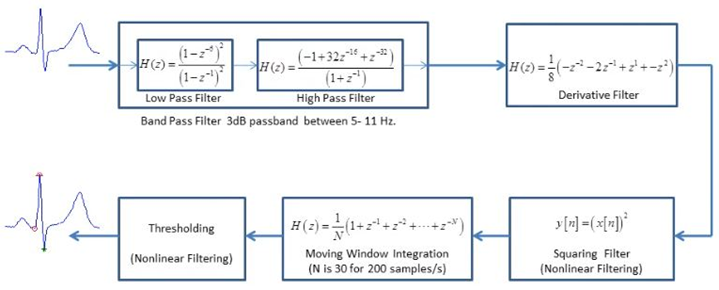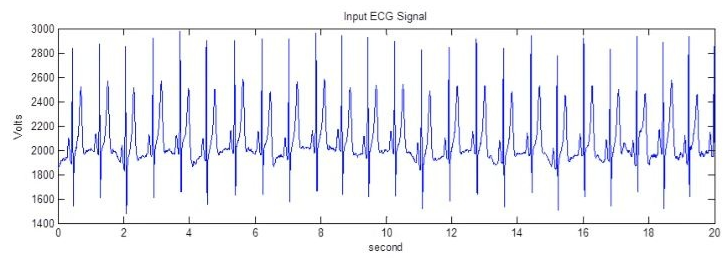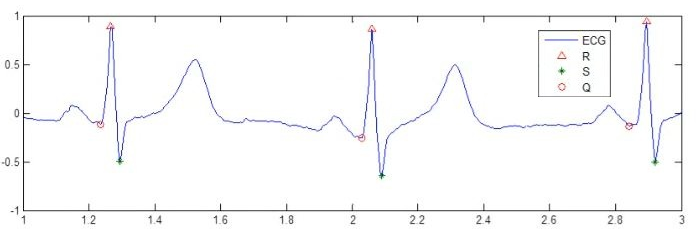The MATLAB code for the QRS Detection program and also the sample ECG files which are used by the MATLAB code (ECG3.dat, ECG4.dat, ECG5.dat, ECG6.dat).

I have added a lot of comments in the code (before each step) so that it is understandable.

We have taken the input signal as an ECG signal from the MIT-BIH Arrhythmia Database ("http://physionet.ph.biu.ac.il/physiobank/database/mitdb/")

The sequence of filtering operations in the algorithm have been indicated below:

SEQUENCE OF FILTERING OPERATIONSSo, in short, the algorithm accepts an input ECG signal and outputs the QRS complex wave, as shown below:

INPUT OF THE SYSTEMOUTPUT OF THE SYSTEMOnce the QRS Wave has been detected from the input ECG signal (as shown above), it can be used for detection of cardiac abnormalities using the following table (from Wikipedia):